# NOW Function in Excel

This function is categorized as a Date and Time function that returns the current date and time in Excel.Reviewed by

Christopher Haynes

Expertise: Asset Management | Investment Banking

Updated:

September 18, 2022

If you have read our article on the TODAY function, you will know that it returns the current date. So, if today's date is 04/02/2022 and you use the formula =TODAY() in the spreadsheet, the result you will get is 04/02/2022.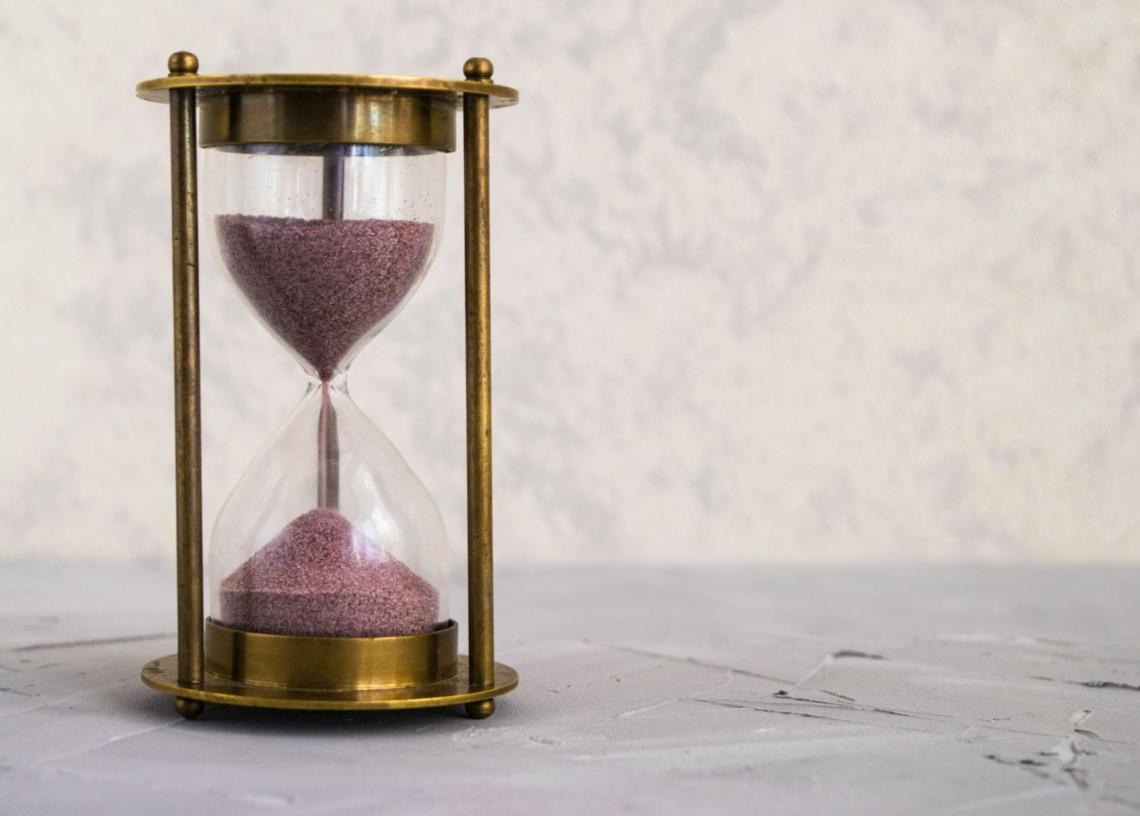The NOW function takes it one step further and is an extension of the TODAY function. How does it take the result one step further?

It returns the current date along with the current Time in Excel, which, in simple terms, is called a timestamp.

## What is the NOW() function?

You usually call your friends and ask them," Hey, what are you doing?" When we say that, we mean at that exact moment. If that moment is considered in terms of date and time, we get the result from the NOW() function.

The NOW() function is categorized as a Date and Time function that returns the current date and Time in Excel.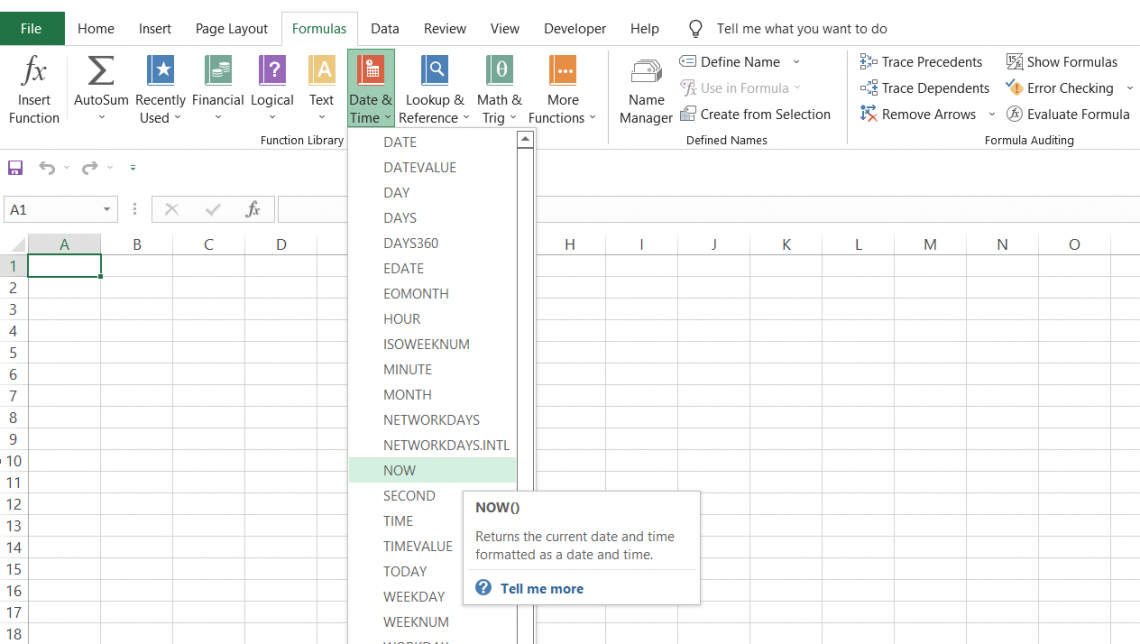It isn't effortless to remember the day and time you made a phone call to your friend. Then, the call history comes in, which works on the same principle as the NOW() function. So, for example, it will store the call time as 2nd April 2022, at 11:12 pm.

Similarly, in Excel, you can use the NOW() function to save instances of Time as and when you work on a particular project or while working on essential reports.

Since it is a volatile function, saving and closing Excel and reopening it after some time will automatically refresh the result from the Excel formula. The part represents the date and time in MM DD YYYY HH: MM format, where the Time is displayed in a 24-hour format.

## Syntax

The syntax for the function is not rocket science. You don't need to add any additional arguments. You begin with the equals (=) sign, followed by the function name, and end the formula with parenthesis (round brackets) - That's it!

So, if you use the formula as =NOW() in Excel, the result in the spreadsheet will be: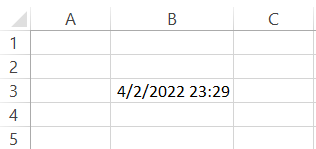Even though the Time is displayed in the HH: MM format, the seconds are still counted behind the scenes, automatically updating the Time whenever you open the file.

If the Time does not automatically update, you have selected the option to switch off automatic calculations in Excel. You can enable it by going into 'Options' and then into Calculation options and setting Workbook Calculation to 'Automatic.'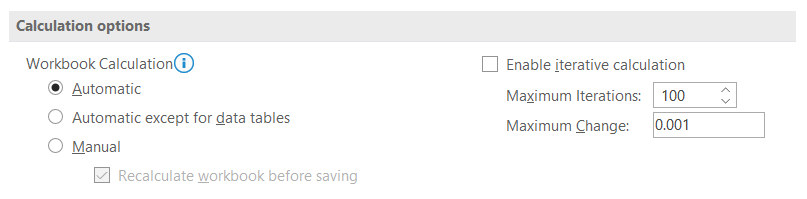You may prefer the 'Manual' mode for calculations, which is useful when working on large amounts of data, and you want to avoid Excel slowing down due to repetitive measures. In this case, just press F9, and the spreadsheet data will refresh.

## Date and Time as a static, unchangeable value

"The Time and date change every Time we open Excel! How do we maintain an hourly task tracker if all the timestamps automatically update to the current Time?"

As we said, NOW() is a highly volatile function and tends to change value when you open or close the file.

Is there an alternative? Yes, and it is pretty simple as well. The option can be broken down into two parts:

• Date - To get a static date, use the keyboard key combination of Ctrl + semicolon key (;). This will give you the current date in Excel, similar to hard coding the value.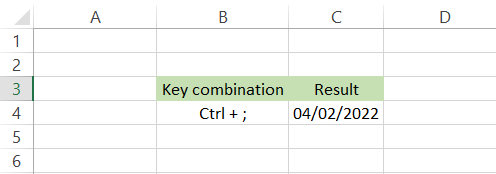• Time - You can get the current Time by using the key combination of Ctrl + Shift + semicolon key(;).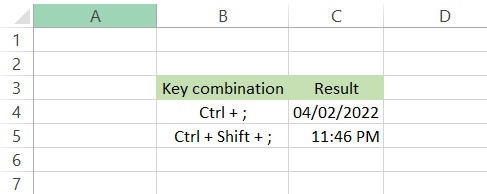What next? It is not efficient if we write them both in separate cells. So, click on cell C6 and first use the key combination for the date, followed by 'space' and then the key combination for Time.

The final static result that you will get in the spreadsheet is illustrated below: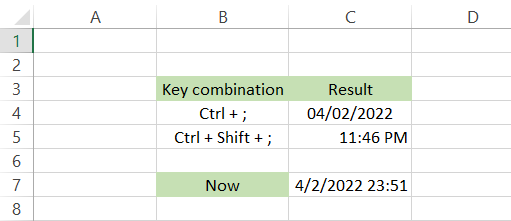You can open the file a thousand times and close it a thousand times more; still, the date and Time would not change in the spreadsheet.

## Changing the date and time format

If you receive a file from an external source, there is a high probability that the date and Time will be in entirely different formats.

It isn't easy to compare different times and dates and perform comparative analyses if such is the case. So, if you need the result of your NOW() function or any other dates in a similar format, select the cell and press Ctrl + 1.

This will open up the format cells dialog box: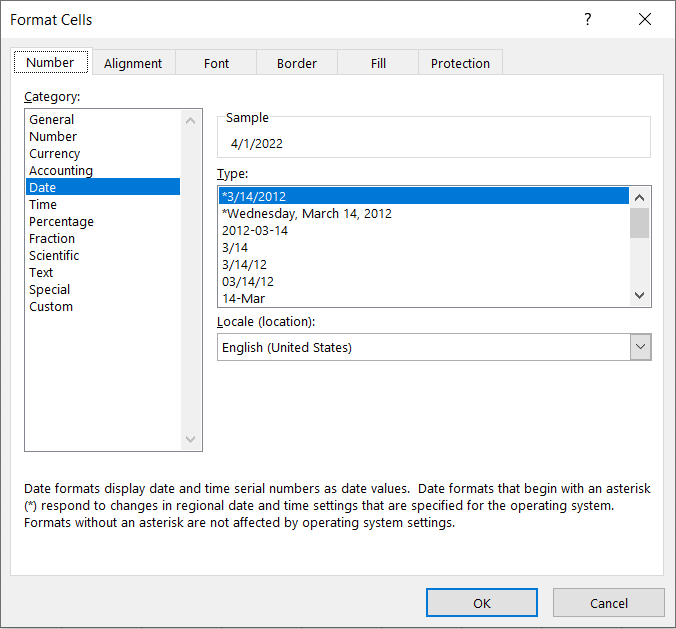Since this is a combination of date and Time, you will need to create a custom format using references from the 'Date' and 'Time' categories, respectively.

We went with the dd-mmm-yy format for the current date and hh:mm: ss for the Time. So even though, by default, the function only displays the hours and minutes, you can show the seconds by changing the format.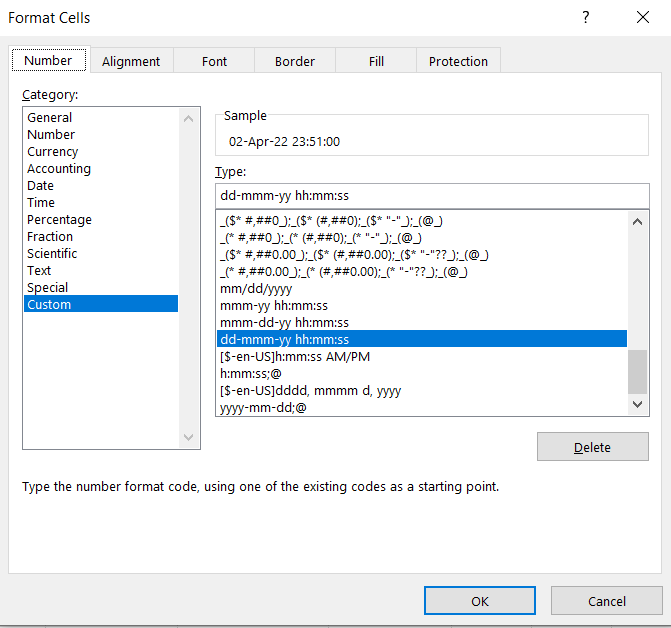Once you set up the desired format in the 'Custom' category, click on OK. The spreadsheet should look as illustrated below: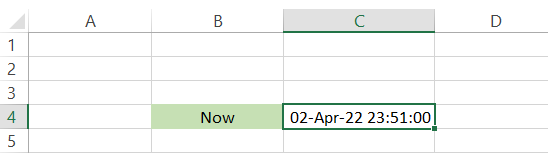Begin your journey into Excel modeling with our free Excel Modeling Crash Course.

## Different ways to use the function

Similar to the TODAY() function, you can use the NOW() function to find the difference between the current date and Time and a particular date and Time in the past or future.

You can also find a particular date by adding or subtracting the 'n' number of days.

On top of that, you can combine it with other functions that help you to complete different objectives.

### Finding the difference between NOW() and a particular date and Time

Suppose that you made a task tracker for one of your projects. The clients need to know how long you have worked on the project so that they can process the current paycheck for you. The current date/time and the starting date/time for the project are as illustrated below: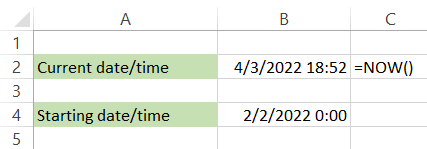Subtract the two cells, i.e., B2 - B4, to give you the following result in cell B6: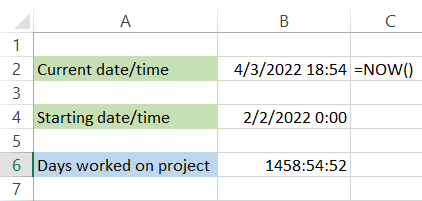We have changed the format to [h]:mm: ss, which gives us 1458:54:52 as the difference between the two dates. Putting the hours inside the square brackets enables us to override the 24 hr clock and display the hours as per the difference between the two dates.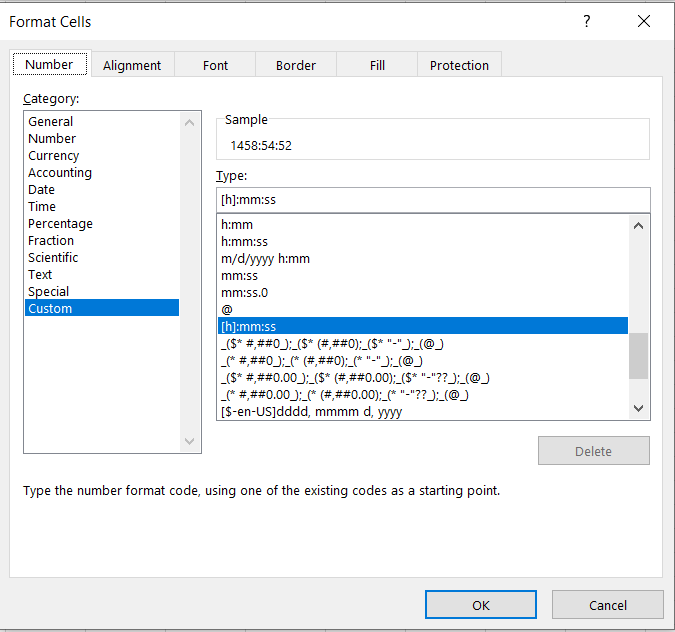If you don't want the result in hours, all you need to do is use a combination of the CONCATENATE, INT, and TEXT functions such that the formula is

=CONCATENATE(INT(B6)," days, "TEXT(B6, "HH: MM")).

This will return 60 days, 19 hours, and 56 mins spent working on the project.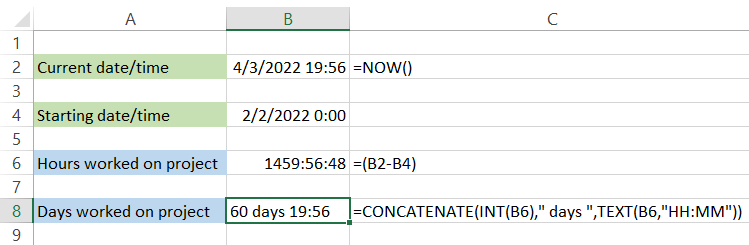We know you may be wondering - Why go to such lengths, using different functions, to calculate the days along with the Time? Why not just format the cells so it represents the date and Time together?

Well, if you select cell B6 and go to format cells and type in different custom formats that include days as 'd' or 'dd,' you won't get the correct result since a 'day' parameter will only store up to 31 days.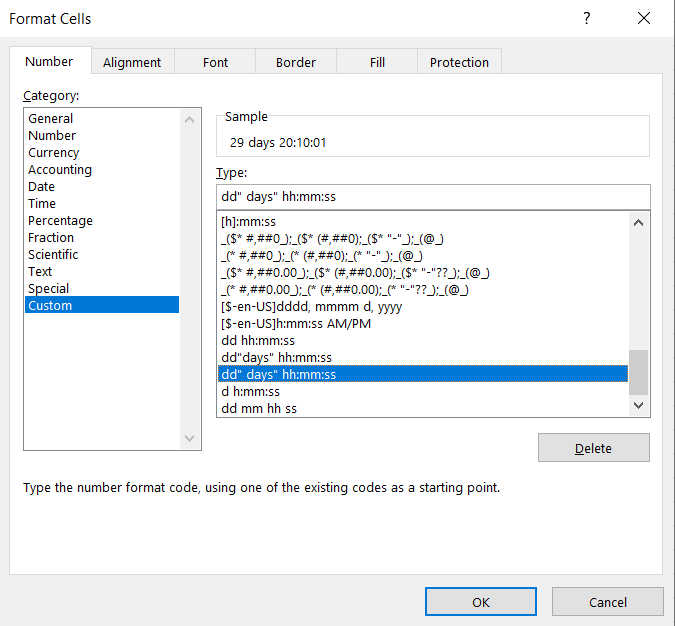You can probably express the result in a fraction or decimal point, which is closer to the answer we expect but does not pinpoint the exact result.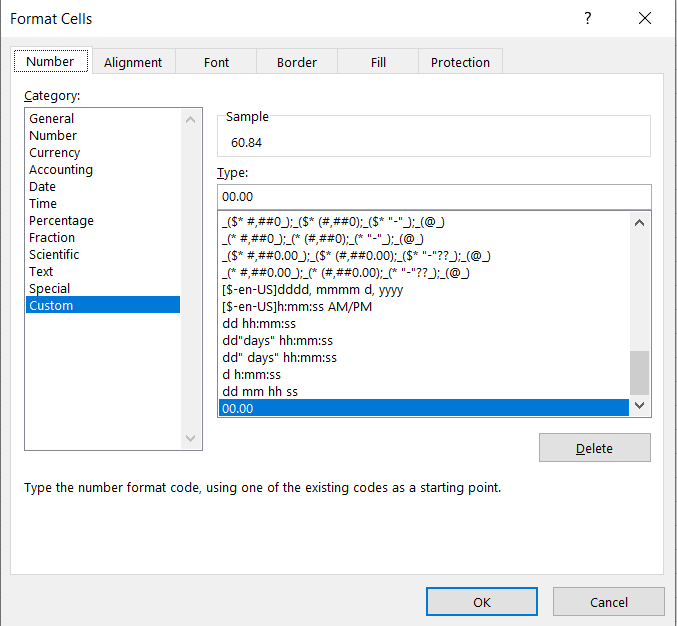Hence, if the hour's format works, you can select it from the Format Cells dialog box or use the formula to calculate the result in days:hours: minutes format.

Similarly, if it's a date in the future, as illustrated below, all you need to do is subtract B4 from B2.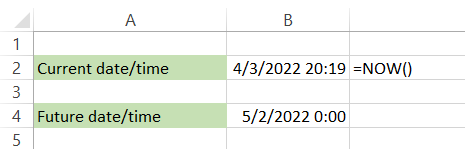This will give you the hours in [h]:mm: ss as 675:40:37. Alternatively, by using the formula

=CONCATENATE(INT(B6)," days, "TEXT(B6, "HH: MM"))

you can convert it to days:### Finding a date in the past or future

Finding a date in the past or future from the 'current date and Time is relatively easy. All you need to do is add the number of days to the function, and it will automatically calculate the past or future date.

Let's say you book an appointment at your dentist around 6:00 pm, and the receptionist schedules the visit in one week. The data will look as illustrated below: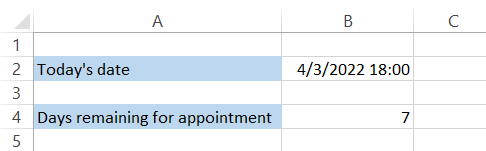All you have to do is add cells B2 and B4 to get the following result: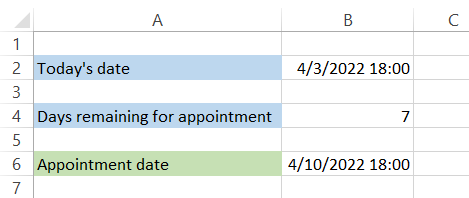But what if the receptionist does not schedule the appointment at the same time, say, 6:00 pm?

Well, one of the ways you can get the expected result is by using a combination of the CONCATENATE and TEXT functions.

Assume that the receptionist says that your appointment is scheduled at 3:00 pm in a week, as illustrated below: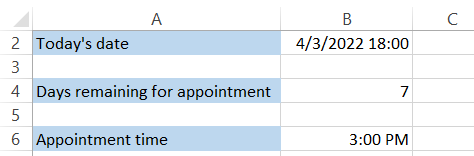The formula that you will use to get the final result is

=CONCATENATE(TEXT(B2+B4, "mm-dd-yyyy"),TEXT(B6," HH:MM"))

which will add today's date, add 7 days to it, and, finally, concatenate the appointment time to the final result.

You will get the following result in the spreadsheet: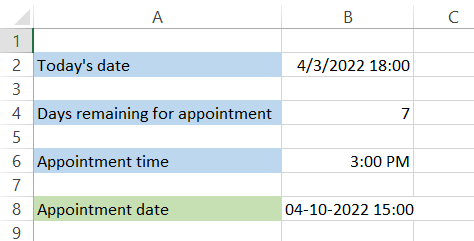Similarly, you can find a date in the past by subtracting the number of days that have since passed.### Excluding weekends from the calculations

If you want to exclude Saturdays and Sundays when finding a date in the past or future, you can use the WORKDAY function. This can be helpful in real-life scenarios to communicate deadlines to clients based on working days.

So, if your clients say, "Hey, can you get this project done in 21 working days by 3:30 pm EST ?" All you need to do is head over to Excel and use the formula

=WORKDAY(NOW(),21)+TEXT("3:30 pm", "HH: MM")

to get the following result: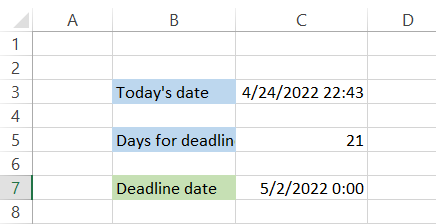Since we used the WORKDAY function, the Time resets to 12:00 am, so you need to separately add the deadline time using the formula TEXT("3:30 pm", "HH:MM"). Had you ignored this part of the formula, you would have gotten the following result: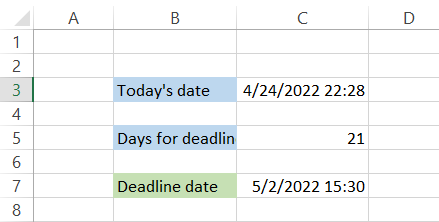There is a significant difference in the time component for the deadline date. Also, what would the deadline date have been if we had included weekends in our calculations?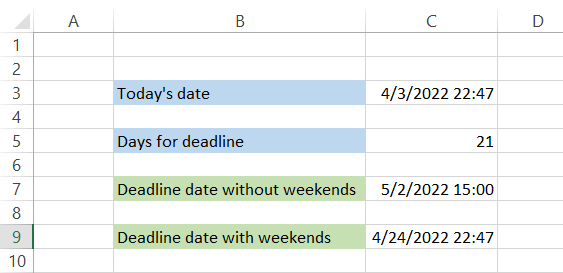As you can see, there is a significant difference in the date when weekends are included or excluded from our calculations.

Also, we do not have to add the time component to our result. We need to add cells C3 and C5 to get 4/24/2022 at 22:47.

If you wish to change the deadline time, use the formula

=CONCATENATE(TEXT(C3+C5, "mm-dd-yyyy"),TEXT("3:30 PM" HH:MM"))

to get the following result: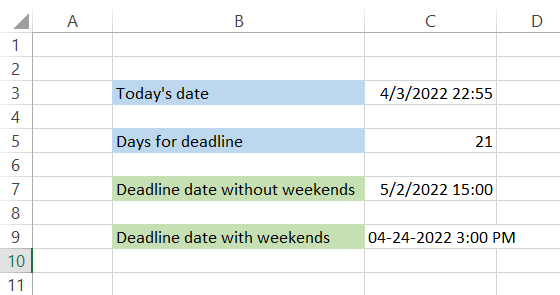## Practical Example #1

Please assume that the students in a school must submit their homework every Saturday. Therefore, the data is illustrated below: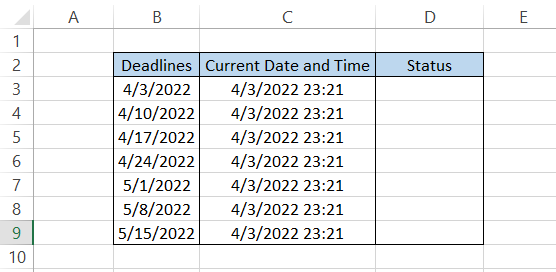If you need to check the status of whether the student can still submit their assignments, you must use a combination of two functions - IF and NOW. The formula that you will use in cell D3 is =IF(NOW()>B3, "Past Deadline," "Due") to get the following asset: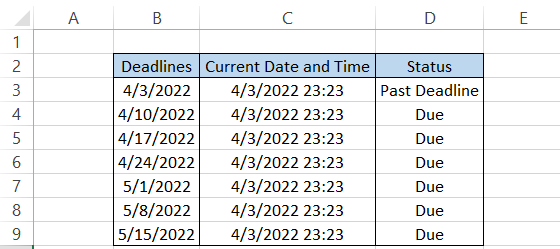The formula essentially checks if the deadline day has passed by comparing it with the current date and Time in column C. If the current date is more significant, you will get the result as "Past Deadline"; otherwise, you can still submit your assignment.

## Practical Example #2

The combination of NOW and TIME functions works wonders if you need to determine how long it will take you to travel from point A to B. Assume that you were on a road trip with your friends and need to travel back home from Chicago to New York.

If the distance between the two points is 780 km and you will be traveling at an average speed of 40 km/hr, it will take 19.5 hours to travel back home using the simple calculation of Time = Distance/Speed.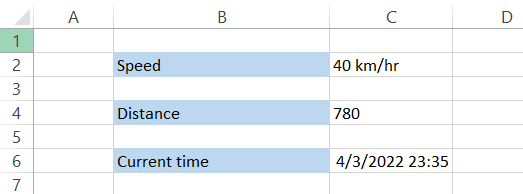Instead of calculating the components separately, you can get the final result in just one go. For example, assuming you begin your journey on 3rd April 2022, at 11:36 pm, around midnight, you can find the expected arrival date by using the formula =NOW()+TIME((780/40),0,0) to get the following result: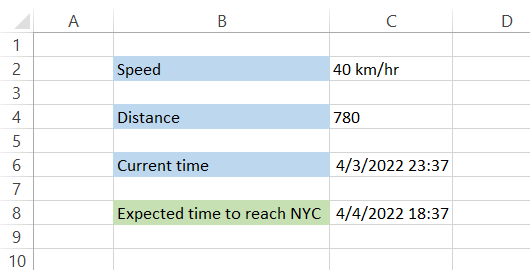You will arrive in New York around 6:37 pm on 4th April 2022.

## Practical Example #3

Need a date/time tracker that automatically updates the timestamp in a spreadsheet if you edit a value in a particular column? Use the VBA code below in Excel if you are working on a critical project and need to know when you made specific changes.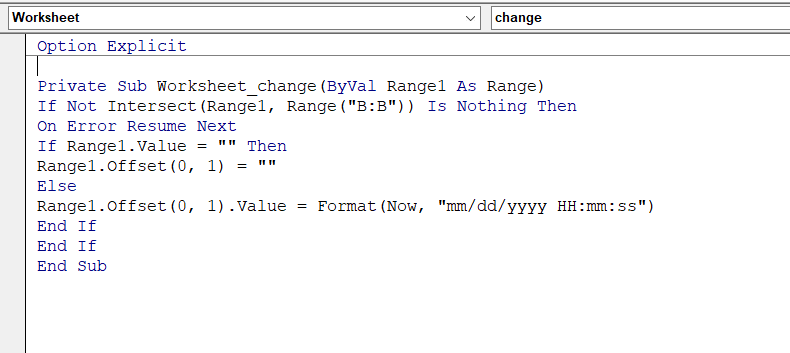As you can see below, if we type a particular status in column B (the timestamp will only be added if the cells in column B are filled), the cell offsets, and we can find the current date and Time in column C.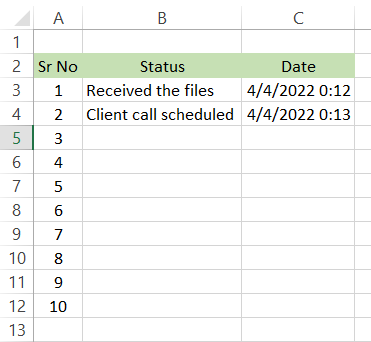There are two important things to remember before using the VBA code:

• First, it is a worksheet dedicated code that will not work on any other spreadsheet in your Excel workbook. If you need it to work on another dedicated spreadsheet, copy and paste the code into the respective sheet.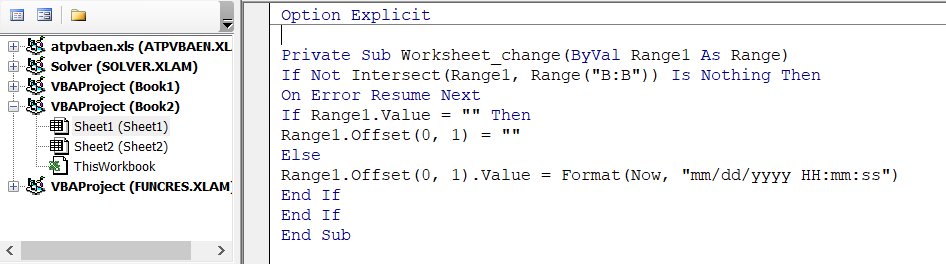As you can see, we have used Sheet1 for the VBA code.

• You cannot begin with Sub..End Sub for this code to run. When you open the Visual Basics for applications to write the code on Sheet1, you first need to select the object as 'Worksheet' instead of (General).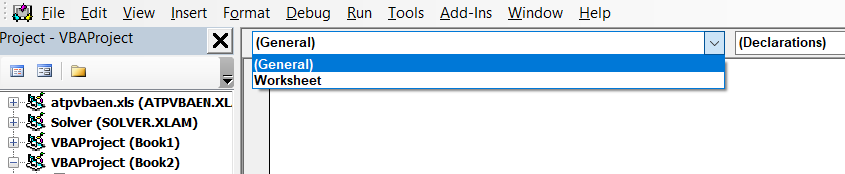You will find another dropdown with 'Declarations,' where you need to select 'Change.' Once selected, the VBA window should look like this: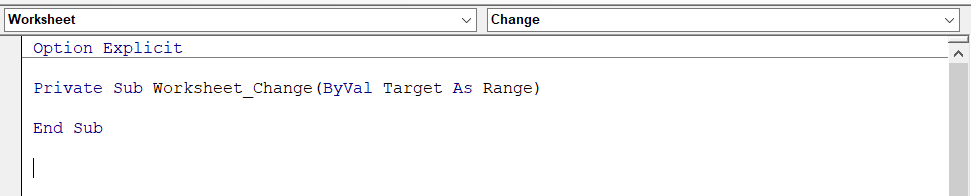You can write the VBA code, and you are good to go!

## Important points to remember

• NOW() function represents the result in mm/dd/yyyy hh:ss format.
• The function will return the result based on the date and time in your laptop/computer system. If the system displays the wrong date and Time, the result will also be wrong.
• As NOW() is a volatile function, the date and Time may change the next time you open the Excel file. To avoid changes, hard code the values using the date shortcut Ctrl + Apostrophe key(;), and time shortcut Ctrl + Shift + Apostrophe key(;).### Everything You Need To Master Excel Modeling

To Help You Thrive in the Most Prestigious Jobs on Wall Street.

Researched and Authored by Akash Bagul | Linkedin

Reviewed and edited by James Fazeli-Sinaki | LinkedIn